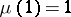# Möbius function

(diff) ← Older revision | Latest revision (diff) | Newer revision → (diff)
An arithmetic function of natural argument:,ifis divisible by the square of a prime number, otherwise, whereis the number of prime factors of the number. This function was introduced by A. Möbius in 1832.
The Möbius function is a multiplicative arithmetic function;if. It is used in the study of other arithmetic functions; it appears in inversion formulas (see, e.g. Möbius series). The following estimate is known for the mean value of the Möbius function :whereis a constant. The fact that the mean value tends to zero asimplies an asymptotic law for the distribution of prime numbers in the natural series.